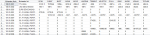# SQL SorguAylık Hizmet Kart Dökümü

#### Murat OSMA

Yönetici
Site Yöneticisi
Logo'da Aylık Hizmet Kart Dökümü için bu sorguyu kullanabilirsiniz.

SQL:
``````SELECT
[Muhasebe Kodu],
sum(OCAK) as OCAK,SUM(SUBAT) AS SUBAT,SUM(MART) AS MART,SUM(NISAN)AS NISAN,
SUM(MAYIS)AS MAYIS,SUM(HAZIRAN)AS HAZIRAN,SUM(TEMMUZ)AS TEMMUZ,SUM(AGUSTOS)AS AGUSTOS,
SUM(EYLUL)AS EYLUL,SUM(EKIM)AS EKIM,SUM(KASIM)AS KASIM, SUM(ARALIK)AS ARALIK, SUM(TOPLAM)AS TOPLAM
from ( select M.DEFINITION_ AS [Muhasebe Adı],
M.code [Muhasebe Kodu],
CASE WHEN MONTH(EMF.DATE_)<=1 THEN (CASE WHEN EMF.TRCODE IN(1,2,3,4,5,6,8,10) THEN SUM(EMF.DEBIT-EMF.CREDIT) END) ELSE 0 END AS OCAK,
CASE WHEN MONTH(EMF.DATE_)<=2 THEN (CASE WHEN EMF.TRCODE IN(1,2,3,4,5,6,8,10) THEN SUM(EMF.DEBIT-EMF.CREDIT)END) ELSE 0 END AS SUBAT,
CASE WHEN MONTH(EMF.DATE_)<=3 THEN (CASE WHEN EMF.TRCODE IN(1,2,3,4,5,6,8,10) THEN SUM(EMF.DEBIT-EMF.CREDIT) END) ELSE 0 END AS MART,
CASE WHEN MONTH(EMF.DATE_)<=4 THEN (CASE WHEN EMF.TRCODE IN(1,2,3,4,5,6,8,10) THEN SUM(EMF.DEBIT-EMF.CREDIT) END) ELSE 0 END AS NISAN,
CASE WHEN MONTH(EMF.DATE_)<=5 THEN (CASE WHEN EMF.TRCODE IN(1,2,3,4,5,6,8,10) THEN SUM(EMF.DEBIT-EMF.CREDIT) END) ELSE 0 END AS MAYIS,
CASE WHEN MONTH(EMF.DATE_)<=6 THEN (CASE WHEN EMF.TRCODE IN(1,2,3,4,5,6,8,10) THEN SUM(EMF.DEBIT-EMF.CREDIT) END) ELSE 0 END AS HAZIRAN,
CASE WHEN MONTH(EMF.DATE_)<=7 THEN (CASE WHEN EMF.TRCODE IN(1,2,3,4,5,6,8,10) THEN SUM(EMF.DEBIT-EMF.CREDIT) END) ELSE 0 END AS TEMMUZ,
CASE WHEN MONTH(EMF.DATE_)<=8 THEN (CASE WHEN EMF.TRCODE IN(1,2,3,4,5,6,8,10) THEN SUM(EMF.DEBIT-EMF.CREDIT) END) ELSE 0 END AS AGUSTOS,
CASE WHEN MONTH(EMF.DATE_)<=9 THEN (CASE WHEN EMF.TRCODE IN(1,2,3,4,5,6,8,10) THEN SUM(EMF.DEBIT-EMF.CREDIT) END) ELSE 0 END AS EYLUL,
CASE WHEN MONTH(EMF.DATE_)<=10 THEN (CASE WHEN EMF.TRCODE IN(1,2,3,4,5,6,8,10) THEN SUM(EMF.DEBIT-EMF.CREDIT) END) ELSE 0 END AS EKIM,
CASE WHEN MONTH(EMF.DATE_)<=11 THEN (CASE WHEN EMF.TRCODE IN(1,2,3,4,5,6,8,10) THEN SUM(EMF.DEBIT-EMF.CREDIT)END) ELSE 0 END AS KASIM,
CASE WHEN MONTH(EMF.DATE_)<=12 THEN (CASE WHEN EMF.TRCODE IN(1,2,3,4,5,6,8,10) THEN SUM(EMF.DEBIT-EMF.CREDIT) END) ELSE 0 END AS ARALIK,
CASE WHEN MONTH(EMF.DATE_) BETWEEN 1 AND 12 THEN (CASE WHEN EMF.TRCODE IN(1,2,3,4,5,6,8,10) THEN SUM(EMF.DEBIT-EMF.CREDIT) END) ELSE 0 END AS TOPLAM
FROM LG_006_EMUHACC M INNER JOIN LG_006_01_EMFLINE EMF
ON EMF.ACCOUNTCODE=M.CODE
WHERE  M.ACTIVE=0 AND EMF.CANCELLED=0
GROUP BY
M.code,M.DEFINITION_,EMF.TRCODE,MONTH(EMF.DATE_)) as tablom
ORDER BY [Muhasebe Kodu]``````

Sonuç TabloSon düzenleme:

Yeni Üye
TEŞŞEKKÜRLER

Yeni Üye
teşekkürler

Yeni Üye

İsimsiz
TEŞKKÜRLER

Yeni Üye
Teşekkürler

Yeni Üye
Teşekkür ederim

Yeni Üye
Teşekkürler

Yeni Üye
Teşekkürler